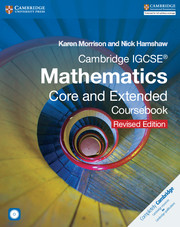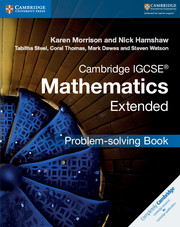Cart

\$50.02

Availability: In stock

× This is a Sterling guide price. You will be invoiced in the appropriate currency for your country.
• ISBN:9781316629703
• Format:Print/online bundle
• Subject(s):Mathematics
• Qualification:Cambridge IGCSE
• Author(s):Karen Morrison, Nick Hamshaw
• Available from: February 2017

Revised edition of the IGCSE Mathematics Core and Extended Coursebook for the 0580 syllabus for examination from 2015.

\$50.02

Availability: In stock

× This is a Sterling guide price. You will be invoiced in the appropriate currency for your country.

Send a Query

formRow
×
• DESCRIPTION

The Cambridge IGCSE® Mathematics Core and Extended Coursebook plus IGCSE Mathematics Online offers students and teachers a combination of support resources for the Cambridge IGCSE® Mathematics syllabus (0580).The coursebook provides clear explanations and practice exercises to help students master mathematical skills. IGCSE Mathematics Online offers additional lessons with explanations, extra questions for students to tackle, worksheets and walkthroughs.IGCSE Mathematics Online allows teachers to set classroom and homework activities to individual students or entire classes. It contains a test-generator, with a marking and recording system to help track student progress.To start your free trial today please visit: https://igcsemaths.cambridge.org/home/freeTrial

• Contents
• FEATURES
• Detailed coursebook with clear explanations and practice exercises alongside an intuitive digital resource offers students a comprehensive resource to give them confidence when learning mathematics.

• Numerous reinforcement exercises, additional worksheets and exam-preparation tasks give students confidence in their skills.

• Organising classroom and homework activities is easy for teachers using the intuitive IGCSE Mathematics Online interface and result tracker feature.

• Free trial
• CONTENTS
• COURSEBOOK: Unit 1: 1. Reviewing number concepts
• 2. Making sense of algebra
• 3. Lines, angles and shapes
• 4. Collecting, organising and displaying data
• Unit 2: 5. Fractions
• 6. Equations and transforming formulae
• 7. perimeter, area and volume
• 8. Introduction to probability
• Unit 3: 9. Sequences and sets
• 10. Straight lines and quadratic equations
• 11. Pythagoras' theorem and similar shapes
• 12. Averages and measures of speed
• Examination Practice: structured questions for Units 1-3
• Unit 4: 13. Understanding measurement
• 14. Further solving of equations and inequalities
• 15. Scale drawings, bearings and trigonometry
• 16. Scatter diagrams and correlation
• Unit 5: 17. Managing money
• 18. Curved graphs
• 19. Symmetry and loci
• 20. Histograms and frequency distribution diagrams
• Unit 6: 21. Ratio, rate and proportion
• 22. More equations, formulae and functions
• 23. Transformations and matrices
• 24. Probability using tree diagrams. Examination Practice: structured questions for units 4-6. Exercise answers
• Glossary
• Index. IGCSE Mathematics Online: 1. Reviewing number concepts
• 2. Making sense of algebra
• 3. Lines, angles and shapes
• 4. Collecting, organising and displaying data
• 5. Fractions
• 6. Equations and transforming formulae
• 7. perimeter, area and volume
• 8. Introduction to probability
• 9. Sequences and sets
• 10. Straight lines and quadratic equations
• 11. Pythagoras' theorem and similar shapes
• 12. Averages and measures of speed
• 13. Understanding measurement
• 14. Further solving of equations and inequalities
• 15. Scale drawings, bearings and trigonometry
• 16. Scatter diagrams and correlation
• 17. Managing money
• 18. Curved graphs
• 19. Symmetry and loci
• 20. Histograms and frequency distribution diagrams
• 21. Ratio, rate and proportion
• 22. More equations, formulae and functions
• 23. Transformations and matrices
• 24. Probability using tree diagrams.\$47.64 Cambridge IGCSE Mathematics View product\$94.74 Cambridge IGCSE® Mathematics View product\$26.50 Cambridge IGCSE® Mathematics View product

Not already registered? Create an account now. ×

Other Cambridge websitesNeed a local sales consultant?